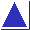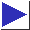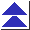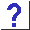# comp.lang.c FAQ list ·Question 13.14

Q: How can I add N days to a date? How can I find the difference between two dates?

A: The ANSI/ISO Standard C mktime and difftime functions provide some (limited) support for both problems. mktime accepts non-normalized dates, so it is straightforward to take a filled-in struct tm, add or subtract from the tm_mday field, and call mktime to normalize the year, month, and day fields (and incidentally convert to a time_t value). difftime computes the difference, in seconds, between two time_t values; mktime can be used to compute time_t values for two dates to be subtracted.

However, these solutions are guaranteed to work correctly only for dates in the range which can be represented as time_t's [footnote] . The tm_mday field is an int, so day offsets of more than 32,736 or so may cause overflow. (See below for an alternative solution without these limitations.) Note also that at daylight saving time changeovers, local days are not 24 hours long, so be careful if you try to divide by 86,400 seconds/day.

Here is a code fragment to compute the date 90 days past October 24, 1994:

```#include <stdio.h>
#include <time.h>

tm1.tm_mon = 10 - 1;
tm1.tm_mday = 24;
tm1.tm_year = 1994 - 1900;
tm1.tm_hour = tm1.tm_min = tm1.tm_sec = 0;
tm1.tm_isdst = -1;

tm1.tm_mday += 90;

if(mktime(&tm1) == -1)
fprintf(stderr, "mktime failed\n");
else	printf("%d/%d/%d\n",
tm1.tm_mon+1, tm1.tm_mday, tm1.tm_year+1900);
```
(Setting tm_isdst to -1 helps to guard against daylight saving time anomalies; setting tm_hour to 12 would, too.)

Here is a piece of code to compute the difference in days between February 28 and March 1 in the year 2000:

```	struct tm tm1, tm2;
time_t t1, t2;

tm1.tm_mon = 2 - 1;
tm1.tm_mday = 28;
tm1.tm_year = 2000 - 1900;
tm1.tm_hour = tm1.tm_min = tm1.tm_sec = 0;
tm1.tm_isdst = -1;

tm2.tm_mon = 3 - 1;
tm2.tm_mday = 1;
tm2.tm_year = 2000 - 1900;
tm2.tm_hour = tm2.tm_min = tm2.tm_sec = 0;
tm2.tm_isdst = -1;

t1 = mktime(&tm1);
t2 = mktime(&tm2);

if(t1 == -1 || t2 == -1)
fprintf(stderr, "mktime failed\n");
else {
long d = (difftime(t2, t1) + 86400L/2) / 86400L;
printf("%ld\n", d);
}
```

Another approach to both problems, which will work over a much wider range of dates, is to use ``Julian day numbers''. A Julian day number is the number of days since January 1, 4013 BC. [footnote] Given ToJul and FromJul routines declared as

```/* returns Julian for month, day, year */
long ToJul(int month, int day, int year);

/* returns month, day, year for jul */
void FromJul(long jul, int *monthp, int *dayp, int *yearp);
```
adding n days to a date can be implemented as
```	int n = 90;
int month, day, year;
FromJul(ToJul(10, 24, 1994) + n, &month, &day, &year);
```
and the number of days between two dates is
```	ToJul(3, 1, 2000) - ToJul(2, 28, 2000)
```
Code for handling Julian day numbers can be found in the Snippets collection (see question 18.15c), the Simtel/Oakland archives (file JULCAL10.ZIP, see question 18.16), and the ``Date conversions'' article mentioned in the References.